# Truncated cone

Calculate the volume of a truncated cone with base radiuses r1=13 cm, r2 = 10 cm and height v = 8 cm.

Result

V =  3342.7 cm3

#### Solution:

$v_2 = \dfrac{r_2}{r_1-r_2}v = \dfrac{ 10}{ 13-10} 8 = 26.67 \ \\ V = V_1-V_2 = \dfrac{1}{3} \pi (r_1^2(v+v_2) - r_2^2v_2) = 3342.7 \ \text{cm}^3 \ \\$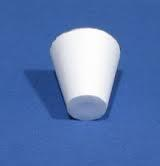Our examples were largely sent or created by pupils and students themselves. Therefore, we would be pleased if you could send us any errors you found, spelling mistakes, or rephasing the example. Thank you!

Leave us a comment of this math problem and its solution (i.e. if it is still somewhat unclear...):Be the first to comment!## Next similar math problems:

1. Rotating coneCalculate volume of a rotating cone with base radius r=12 cm and height h=7 cm.
2. Volume of the coneFind the volume of the cone with the base radius r and the height v. a) r = 6 cm, v = 8 cm b) r = 0,9 m, v = 2,3 m c) r = 1,4 dm, v = 30 dm
3. Volume of cone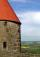Find the volume of a right circular cone-shaped building with a height of 9 cm and a radius base of 7 cm.
4. Cross-sections of a coneCone with base radius 16 cm and height 11 cm divide by parallel planes to base into three bodies. The planes divide the height of the cone into three equal parts. Determine the volume ratio of the maximum and minimum of the resulting body.
5. Cone in cube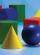The cube is inscribed cone. Determine the ratio of the volume of cone and cube. The ratio express as a decimal number and as percentage.
6. The diagram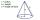The diagram is a cone of radius 8cm and height 10cm. The diameter of the base is. ..
7. Diameter of a cylinderI need to calculate the cylinder volume with a height of 50 cm and a diameter of 30 cm.
8. AverageThe arithmetic mean of the two numbers is 71.7. One number is 5. Calculate the second number.
9. Fire tankHow deep is the fire tank with the dimensions of the bottom 7m and 12m, when filled with 420 m3 of water?
10. Volume of cubeSolve the volume of a cube with width 26cm .
11. Tank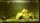The tank bottom has dimensions of 1.5 m and 3 2/6 m. The tank is 459.1 hl of water. How high is the water surface?
12. ClassIn 7.C clss are 10 girls and 20 boys. Yesterday was missing 20% of girls and 50% boys. What percentage of students missing?
13. Seeds 2How many seeds germinated from 1000 pcs, when 23% no emergence?
14. IronIron ore contains 57% iron. How much ore is needed to produce 20 tons of iron?Combine like terms 4c+c-7cThe bicycle pedal gear has 36 teeth, the rear gear wheel has 10 teeth. How many times turns rear wheel, when pedal wheel turns 120x?Solve for x: 3(x + 2) = x - 18